# You Can Multiply Three Numbers in Any Order

Alignments to Content Standards: 5.OA.A 5.MD.C.5

Make sure you have plenty of snap cubes. * Build a rectangular prism that is 2 cubes high, 3 cubes wide, and 5 cubes long. * We will say that the volume of one cube is 1 cubic unit. What is the volume of the rectangular prism? * The volume of the cube is $2\times3\times5$ cubic units. The expression $$2\times(3\times5)$$ can be interpreted as 2 groups with $3\times5$ cubes in each group. $3\times5$ can be interpreted as 3 groups with 5 cubes in each groups. How can you see the rectangular prism as being made of 2 groups with (3 groups of 5 cubes in each)? * Explain how you can see each of these products by looking at the rectangular prism in different ways: $$2\times(5\times3)$$ $$3\times(2\times5)$$ $$3\times(5\times2)$$ $$5\times(2\times3)$$ $$5\times(3\times2)$$

## IM Commentary

The purpose of this task is for students to use the volume of a rectangular prism to see why you can multiply three numbers in any order you want and still get the same result. Formally, this is a consequence of the commutative and associative properties of multiplication. For example, we can see that $$2\times(3\times5)=3\times(5\times2)$$ By using the properties of multiplication step-by-step:

\begin{align} 2\times(3\times5) &= (2\times3)\times5 \text{ (associative property)}\\ &= (3\times2)\times5 \text{ (commutative property)}\\ &= 3\times(2\times5) \text{ (associative property)}\\ &= 3\times(5\times2) \text{ (commutative property)} \end{align}

However, if we just note that in each case we are describing the volume of a rectangular prism with dimensions 2, 3, and 5, then it reinforces the idea that we should be able to multiply in any order, as the volume will be the same regardless of the order in which we name the dimensions. While not one of the field axioms, some people call this the any-order property of multiplication because we can multiply three numbers in any order and get the same product. Students will need snap-cubes, unifix cubes, or cube-shaped blocks for this task. Some students will be able to picture the different decompositions without a physical model, but others will need to build it for themselves to see it, and everyone should have access to these tools the first time they think about this. Later, students can be asked to picture these kinds of decompositions in their head, which can help increase their visualization skills. Note that the task statement doesn't include the other 6 possible expressions we can make when multiplying three numbers. While it is possible to interpret $2\times(3\times5)$ and $(2\times3)\times5$ differently, the difference is pretty subtle and is only worth focusing on if one is trying to explain why it makes sense that multiplication is associative, which this task takes as given. For a task that addresses this directly, please see, 5.MD,OA Using Volume to Understand the Associative Property of Multiplication.

The Standards for Mathematical Practice focus on the nature of the learning experiences by attending to the thinking processes and habits of mind that students need to develop in order to attain a deep and flexible understanding of mathematics. Certain tasks lend themselves to the demonstration of specific practices by students. The practices that are observable during exploration of a task depend on how instruction unfolds in the classroom. While it is possible that tasks may be connected to several practices, only one practice connection will be discussed in depth. Possible secondary practice connections may be discussed but not in the same degree of detail.

This task helps illustrate Mathematical Practice 7, Look for and make use of structure. During this activity, fourth graders are actively connecting the structure of the commutative and associative properties as they decompose a (2x3x5) rectangular prism built with snap cubes into the various groupings such as 2×(3×5), 3×(5×2),  5×(2×3, )or  5×(3×2).  They will realize that regardless of the different decompositions of the rectangular prism the volume of the prism stays the same. This connects to the idea that they can multiply the 3 dimensions of the rectangular prism in any order and the resulting volume will be the same. With experience students should be able to visualize these decompositions in their head.  Through this hands-on exploration of volume, students are immersed in pattern-recognition and pattern-generalizing which not only develops a better understanding of the commutative and associative properties but allows them to apply this understanding to solve other problems.

## Solution

• Here is a picture of such a rectangular prism: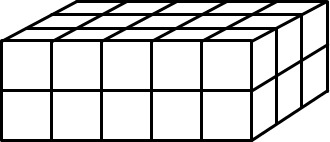• The volume is 30 cubic units. We can break the prism into layers in different ways:

• If we cut horizontally, we will have 2 layers.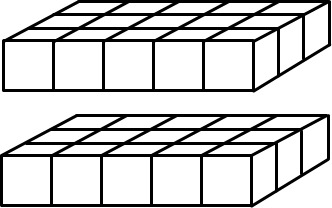Each layer will be a 3 by 5 rectangular solid with a height of 1 unit. We can see a layer as 3 rows with 5 cubic units in each row: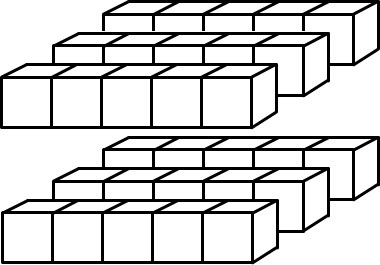So the prism is made up of 2 groups of (3 groups of 5 cubes) which is $2\times(3\times5)$.

• We can also see each layer as 5 columns with 3 cubic units in each column: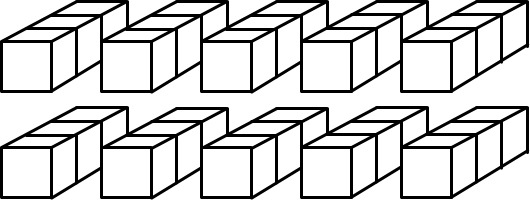So we can see that the prism is made up of 2 groups of (5 groups of 3 cubes) which is $2\times(5\times3)$.

• If we cut vertically along the side that has a length of 3 units, we will have 3 slices with $2\times5$ (or $5\times2$, depending on how you look at it) cubic units in each.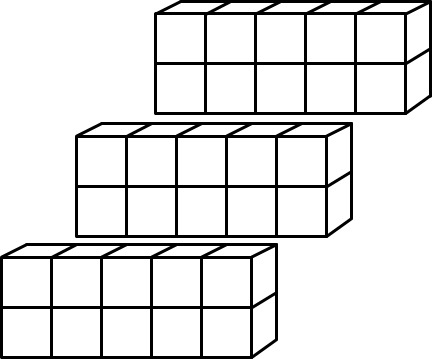• If we cut vertically along the side that has a length of 5 units, we will have or 5 slices with $2\times3$ (or $3\times2$, depending on how you look at it) cubic units in each.This helps show that you can multiply three numbers in any order, because the volume of the same geometric object can be thought of as the product of the three factors taken in any order. Note that the volume doesn't change since the figure doesn't change--we just look at it from different perspectives.